# 06-240/Homework Assignment 9

Just for fun. A certain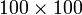$100\times 100$ matrix$A$ of random numbers between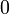$0$ and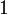$1$ is fed into a computer called Golem, capable of about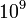$10^9$ arithmetic operations per second (between floating point numbers, at roughly 14 decimal digits of precision).
• Estimate how long it will take Golem to compute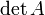$\det A$ using the explicit recursive formula.
• Assuming you are ready to wait and shuffle screens, will you trust the results? (Remember that even if electrical power will be available to eternity and electronic components will never fail, every time a computer adds or multiplies two 14-digit numbers it makes a rounding error of size around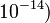$10^{-14})$.
• Estimate how long it will take Golem to compute$\det A$ using row operations.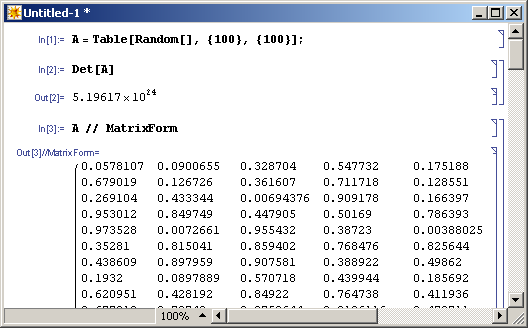Computed on Dror's laptop in a fraction of a second. The matrix is cropped, of course.